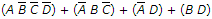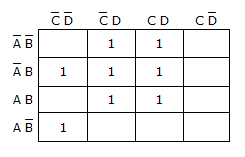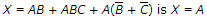# Digital Electronics - Combinational Logic Circuits

### Exercise :: Combinational Logic Circuits - True or False

11.

Even parity is the condition of having an even number of 1s in every group of bits.

 A. True B. False

Explanation:

No answer description available for this question. Let us discuss.

12.

The look-ahead carry method suffers from propagation delays.

 A. True B. False

Explanation:

No answer description available for this question. Let us discuss.

13.

The Boolean equationresults from this Karnaugh map.A. True B. False

Explanation:

No answer description available for this question. Let us discuss.

14.

A pull-up resistor is a resistor used to keep a given point in a circuit HIGH when in the active state.

 A. True B. False

Explanation:

No answer description available for this question. Let us discuss.

15.

The simplified form of.

 A. True B. False